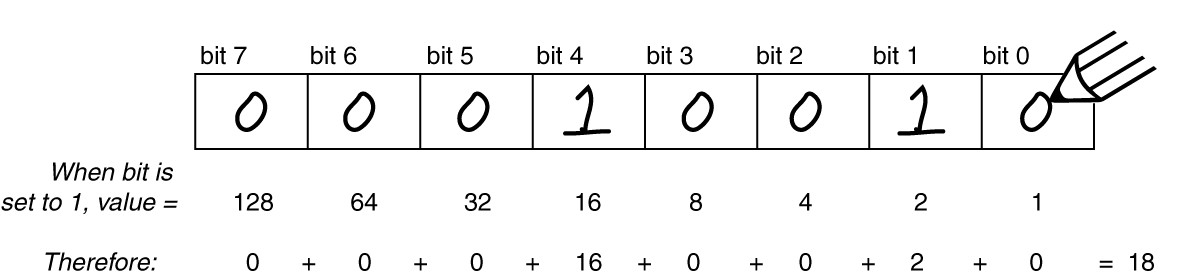Follow

# CV list for version 3.5

Configuration Variables used by V3.5 DecodersCV1 Short decoder address; 1-127 valid
CV2 Start Voltage (useful range 0-100)
CV3 Acceleration rate (each unit = 7mS between speed steps) 255 max.
CV4 Deceleration rate (each unit = 7mS between speed steps) 255 max.
CV5 Vmax, speed at highest speed step. 0=use factory default of 255
CV6 Vmid, speed (on a scale of 1-255) at speed step 7,14,or 63. 0=use default of 127
CV7 Decoder version number. This decoder is 35 which means version 3.5
CV8 Manufactuer ID. NCE = 11 (0B hex)
CV11 Packet timeout value (in ½ second increments) Time the decoder will wait before braking to a
stop after running into a section of track with DC power. 0=Don’t brake
CV15 Decoder programming lock “KEY”. This CV is always programmable even when “locked”
CV16 Decoder programming lock ID. When CV15=CV16, programming is unlocked and the
decoder will respond to programming commands. If CV15 is not equal to CV16 then
decoder programming is locked and it will not program (except CV15) or read.
CV17 High byte of long (4 digit) address
- bit 6,7 always= 1
- bits 0-5 are upper 6 bits of address
CV18 Low byte of long (4 digit) address
CV19 Consist address. (0 or 128 = no consist active)
- bits 0-6 short consist address (1-127 valid)
- bit 7 0= direction is normal, 1= direction is reversed
CV21 Functions active in consist mode. Bit 0 controls F1,bit 1=F2, bit 2=F3, etc.
- bit 0 - 1=function can be controlled at consist address, 0 = no consist control
CV22 Functions active in consist mode. Bits 0,1 control FLF and FLR respectively
each bit 1=function can be controlled at consist address, 0 = no consist control
CV29 - bit 0 1= direction of operation is reversed, 0= direction is normal
- bit 1 1=28 speed mode (always enabled)
- bit 2 1= analog operation mode enabled, 0 = disabled
- bit 4 1= alternate speed table active, 0= use table defined by CV2,5,6
- bit 5 1= use long address in CV17/18, 0= use short address CV1
- bits 3,6,7 are ignored by the decoder
CV30 Set this CV to 2 on the programming track and the decoder will reset to factory settings.
CV33-CV46 function mapping CVs for F0-F12
CV67-CV94 Uploadable speed table steps 1-28 (128 speed mode calculates intermediate steps)
CV95 Reverse trim, values 1-127 add to reverse speed, values 129-255 add to forward speed
CV116 Torque kick rate - number of 16ms periods in a row that motor is ‘kicked’ with voltage pulse
CV117 Torque kick strength - how much voltage is used to kick the motor at slow speeds. Reduces
to 0 as speed is increased.
CV118 Ditch light hold time (in ¼ second increments) after F2 goes off.
CV120-CV128 Effects configuration registers for outputs 1-8